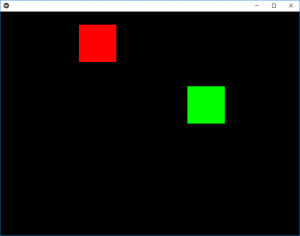# Python | Animation in Kivy using .kv file

Kivy is a platform independent GUI tool in Python. As it can be run on Android, IOS, Linux, and Windows, etc. It is basically used to develop the Android application, but it does not mean that it can not be used on Desktops applications.

## Animation:

Animation and AnimationTransition are used to animate Widget properties. You must specify at least a property name and target value. To use Animation, follow these steps:

• Setup an Animation object
• Use the Animation object on a Widget

To use animation you must have to import:
from kivy.animation import Animation

```Basic Approaches:

1) import runTouchApp
2) import Builder
3) import Widget
4) import Animation
5) import Clock
6) import Window
7) import random
8) import listproperty
9) Build the .kv file
10) Create root class
11) Create the clock and anim react class used to animate the boxes
12) Run the App```

In the below example we are creating the two boxes (red and green) in which we are giving animations to the red box i.e when the App starts the red box is in its animated view but to clear the concepts i am providing the green box which on click provide random animations i.e it goes anywhere at random.
The program consists of two main classes first is clock class which is for the red box as we are declaring it animated and the green box animation is in anim class.

To provide the motion you must have to play with velocity.

Implementation of the Approach:

## Python3

 `# work same as kivy.App used to run the App` `from` `kivy.base ``import` `runTouchApp`   `# to use .kv file as a string we have to import it` `from` `kivy.lang ``import` `Builder`   `# A Widget is the base building block of GUI interfaces in Kivy` `from` `kivy.uix.widget ``import` `Widget`   `# The Clock object allows you to schedule a` `# function call in the future` `from` `kivy.clock ``import` `Clock`   `# Animation and AnimationTransition are` `# used to animate Widget properties` `from` `kivy.animation ``import` `Animation`   `# The Properties classes are used when` `# you create an EventDispatcher.` `from` `kivy.properties ``import` `ListProperty`   `# Core class for creating the default Kivy window. ` `from` `kivy.core.window ``import` `Window`   `# As name suggest used when random things required` `from` `random ``import` `random`     `# load the kv file as string ` `Builder.load_string(``'''` `:`   `# Setting the position (initial) of boxes`   `    ``ClockRect:` `        ``pos: 300, 300` `    ``AnimRect:` `        ``pos: 500, 300`   `# creation and animation of red box` `:` `    ``canvas:` `        ``Color:` `            ``rgba: 1, 0, 0, 1` `        ``Rectangle:` `            ``pos: self.pos` `            ``size: self.size`   `# creation and animation of red box` `:` `    ``canvas:` `        ``Color:` `            ``rgba: 0, 1, 0, 1` `        ``Rectangle:` `            ``pos: self.pos` `            ``size: self.size` `'''``)`     `# Create the root class` `class` `Root(Widget):` `    ``pass`     `# Create the clock class Then is when clicked` `# how much time to animate` `# the red colour block animation is created by it` `class` `ClockRect(Widget):` `    ``velocity ``=` `ListProperty([``10``, ``15``])`   `    ``def` `__init__(``self``, ``*``*``kwargs):` `        ``super``(ClockRect, ``self``).__init__(``*``*``kwargs)` `        ``Clock.schedule_interval(``self``.update, ``1` `/` `60.``)`   `    ``def` `update(``self``, ``*``args):` `        ``self``.x ``+``=` `self``.velocity[``0``]` `        ``self``.y ``+``=` `self``.velocity[``1``]`   `        ``if` `self``.x < ``0` `or` `(``self``.x ``+` `self``.width) > Window.width:` `            ``self``.velocity[``0``] ``*``=` `-``1` `        ``if` `self``.y < ``0` `or` `(``self``.y ``+` `self``.height) > Window.height:` `            ``self``.velocity[``1``] ``*``=` `-``1`     `# Create the Animation class` `# And add animation` `# green colour box is animated through this class` `class` `AnimRect(Widget):` `    `  `    ``def` `anim_to_random_pos(``self``):` `        ``Animation.cancel_all(``self``)` `        ``random_x ``=` `random() ``*` `(Window.width ``-` `self``.width)` `        ``random_y ``=` `random() ``*` `(Window.height ``-` `self``.height)`   `        ``anim ``=` `Animation(x ``=` `random_x, y ``=` `random_y,` `                         ``duration ``=` `4``,` `                         ``t ``=``'out_elastic'``)` `        ``anim.start(``self``)`   `    ``def` `on_touch_down(``self``, touch):` `        ``if` `self``.collide_point(``*``touch.pos):` `            ``self``.anim_to_random_pos()`   `# run the App` `runTouchApp(Root())`

Output:Animated Output video:

Whether you're preparing for your first job interview or aiming to upskill in this ever-evolving tech landscape, GeeksforGeeks Courses are your key to success. We provide top-quality content at affordable prices, all geared towards accelerating your growth in a time-bound manner. Join the millions we've already empowered, and we're here to do the same for you. Don't miss out - check it out now!